# Description of run_ccama.m

For the mass-spring-damper example Matlab function run_ccama.m allows users to:

• choose the number of masses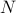• form the dynamic matrix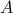;

• form the filter dynamics that generate colored-in-time excitation for the mass-spring-damper system;

• compute the true state covariance matrix of the mass-spring-damper system;

• form the matrix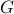of available correlations and the structural identity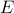;

• choose the low-rank parameter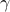;

• choose the optimization parameters through the structured array options;

• call the customized AMA or ADMM algorithms by calling the function ccama.m.

### run_ccama.m

run_ccama
```% Covariance Completion for a mass-spring-damper system
%
% Written by Armin Zare and Mihailo Jovanovic, April 2016
%
% Customized solvers, based on the Alternating Minimization Algorithm (AMA)
% and the Alternating Direction Method of Multipliers (ADMM), are used to
% solve the Covariance Completion problem (CC)
%
% minimize -logdetX + gamma*|| Z ||_*           (CC)
% subject to  A*X + X*A' + Z = 0
%            E.*(C*X*C') - G = 0
%
% A, G, E, L - problem data
% X, Z       - optimization variables
%
```
```clear all
clc
```
```% ==================
% MSD system setup %
% ==================

% number of masses
N = 10;

% identity and zero matrices
I = eye(N);
Ibig = eye(2*N);
ZZ = zeros(N,N);

% forming the state and input matrices
T = toeplitz([2 -1 zeros(1,N-2)]);

% dynamic matrix
A = [ZZ, I; -T, -I];
% input matrix
B = [ZZ; I];
% output matrix
C = Ibig;

% dynamics of the filter that generates colored noise
Af = -I;
Bf = I;
Cf = I;
Df = ZZ;

% form cascade connection of the plant and the filter
Ac = [A, B*Cf; zeros(N,2*N), Af];
Bc = [B*Df; Bf];

% Lyapunov equation for covariance matrix of the cascade systems
% P = [X, Y; Y', Z]
P = lyap(Ac,Bc*Bc');

% Covariance of the state of the plant
Sigma = P(1:2*N,1:2*N);
```
```% =========================================================================
% Structural identity matrix E is formed based on known system correlations
% =========================================================================

% structural identity matrix for known elements of covariance matrix Sigma
E = [I, I; I, I];
% matrix of known output correlations
G = E.*Sigma;
```
```% =================================
% Optimization problem parameters %
% =================================

% low-rank parameter gamma
gamma = 10;

% input options into the optimization procedure
options.rho = 10;
options.eps_prim = 1.e-6;
options.eps_dual = 1.e-6;
options.maxiter = 1.e5;

% initial conditions
Xinit = lyap(A,Ibig);
options.Xinit = Xinit;
options.Zinit = Ibig;
Y1init = lyap(A',-Xinit);
options.Y1init = gamma*Y1init/norm(Y1init,2);
[n, m] = size(C);
options.Y2init = eye(n);
options.method = 1; % AMA
% options.method = 2; % ADMM
```
```% ===================================================================
% optimization procedure -> output = ccama(A,G,E,C,gamma,N,options) %
% ===================================================================

% Call ccama
%
%  Inputs: (1) dynamic matrix A
%              matrix of available output correlations G
%              structural identity matrix E
%              output matrix C
%              low-rank parameter gamma
%              number of masses N
%
%          (2) options
%
%              options.rho      - initial step-size
%              options.eps_prim - tolerance on primal residual
%              options.eps_dual - tolerance on duality gap
%              options.maxiter  - maximum number of AMA iterations
%              options.Xinit    - feasible initial value for matrix X
%              options.Zinit    - feasible initial value for matrix Z
%              options.Y1init   - dual-feasible initial value for Y1
%              options.Y2init   - dual-feasible initial value for Y2
%              options.method   - method = 1, AMA (default)
%                               - method = 2, ADMM
%
%  Outputs: output - structured array containing
%
%           output.X     - matrix X resulting from (CC)
%           output.Z     - matrix Z resulting from (CC)
%           output.Y1    - matrix Y1 resulting from (CC)
%           output.Y2    - matrix Y2 resulting from (CC)
%           output.Jp    - primal objective function at each step
%           output.Jd    - dual objective function at each step
%           output.Rp    - primal residual at each step
%           output.dg    - duality gap at each step
%           output.steps - number of steps for solving (CC)
%           output.time  - cumulative solve time (in seconds) per outer iteration
%           output.flag  - flag = 0, iteration counter reaches its max
%                          flag = 1, problem is solved before maxiter steps
%

tic
output = ccama(A,C,E,G,gamma,n,m,options);
time = toc;
```